CHIRAG GOYAL — Published On June 21, 2021 and Last Modified On June 22nd, 2021

This article was published as a part of the Data Science Blogathon

## Introduction

This article is part of an ongoing blog series on Natural Language Processing (NLP). In the previous article of this series, we completed the statistical or frequency-based word embedding techniques, which are pre-word embedding era techniques. So, in this article, we will discuss the recent word-era embedding techniques.

NOTE: In recent word-era embedding, there are many such techniques but in this article, we will discuss only the Word2Vec technique, which is the most used and popular technique from all of the techniques.

This is part-6 of the blog series on the Step by Step Guide to Natural Language Processing.

2. Recap of Word Embedding

3. What is Prediction-based Embedding?

4. Different Model Architectures for Word representation

• FeedForward Neural Net Language Model (NNLM)
• Recurrent Neural Net Language Model (RNNLM)

5. What is Word2Vec Model?

6. Different algorithms included in Word2Vec

• Continuous Bag of Words (CBOW)
• Skip Gram

7. Project Idea to use Word Embeddings for Text Classification

To follow this part of this blog series properly, you have a good knowledge of the following topics:

• How does an artificial neural network work?
• An in-depth idea of mechanisms by which weights in a neural network are updated (Backpropagation).

If you are not familiar with the above-mentioned concepts, then I would suggest you go through this awesome article to gain an in-depth intuition about neural networks.

For Gradient-based Optimizers, you can refer to the .

Or, if you have an idea of all these topics, then you can refer to this link to check your knowledge.

## Recap of Word Embedding

Word embedding is a way of representing words as vectors. The main goal of word embedding is to convert the high dimensional feature space of words into low dimensional feature vectors by preserving the contextual similarity in the corpus.

These models are widely used for all NLP problems. It first generates a vocabulary with the help of a training corpus and then learns the word embedding representations. In simple words, these models take a text corpus as input and produce the word vectors as output.

They can be used as feature vectors for the Machine Learning model, used to measure text similarity using cosine similarity techniques, words clustering, and text classification techniques, which we will be discussed in the subsequent part of this series.

## Prediction-based Word Embedding

So far, we have discussed the deterministic methods to determine vector representation of the words but these methods proved to be limited in their word representations until the new word embedding technique named word2vec comes to the NLP community.

The popular pre-trained models to create word embedding of a text are as follows:

### Why these models are called Prediction-based?

1. These methods were prediction-based as they give the probabilities to the words.

2. They proved to be state of the art for tasks like word analogies and word similarities.

3. They were also able to achieve algebraic operations tasks such as King -man +woman = Queen, which was considered a result almost magical.

In this article, we will discuss only the word2vec model that is used in today’s era to generate word vectors.

## Different Model Architectures for Word representation

The following model architectures are used for word representations with an objective to maximize the accuracy and minimize the computation complexity:

• FeedForward Neural Net Language Model (NNLM)
• Recurrent Neural Net Language Model (RNNLM)

For training of the above-mentioned models, we use Stochastic gradient descent as an optimizer and backpropagation.

### FeedForward Neural Net Language Model (NNLM)

This model consists of the following layers:

• Input layer,
• Projection layer,
• Hidden layer, and
• Output layer.

This architecture becomes complex for computation between the projection and the hidden layer, as values in the projection layer are dense.

### Recurrent Neural Net Language Model (RNNLM)

This model can efficiently represent more complex patterns than the shallow neural network. This model consists of the following layers:

• Input layer,
• Hidden layer, and
• Output layer.

To train these models for huge datasets, we will be using a large-scale distributed framework known as DistBelief, which would give better results.

### Problem with these models

These models outperform for the huge dataset of words but the main problem in these models is the computation complexity. So, to overcome the computation complexity, the Word2Vec uses CBOW and Skip-gram architecture in order to maximize the accuracy and minimize the computation complexity.

## What is Word2Vec Model?

Word2Vec model is used for Word representations in Vector Space which is founded by Tomas Mikolov and a group of the research teams from Google in 2013. It is a neural network model that attempts to explain the word embeddings based on a text corpus.

These models work using context. This implies that to learn the embedding, it looks at nearby words; if a group of words is always found close to the same words, they will end up having similar embeddings.

To label how words are similar or close to each other, we first fix the window size, which determines which nearby words we want to pick.

For Example, For a window size of 2, implies that for every word, we’ll pick the 2 words behind and the 2 words after it. Let’s see the following example:

`Sentence: the pink horse is eating`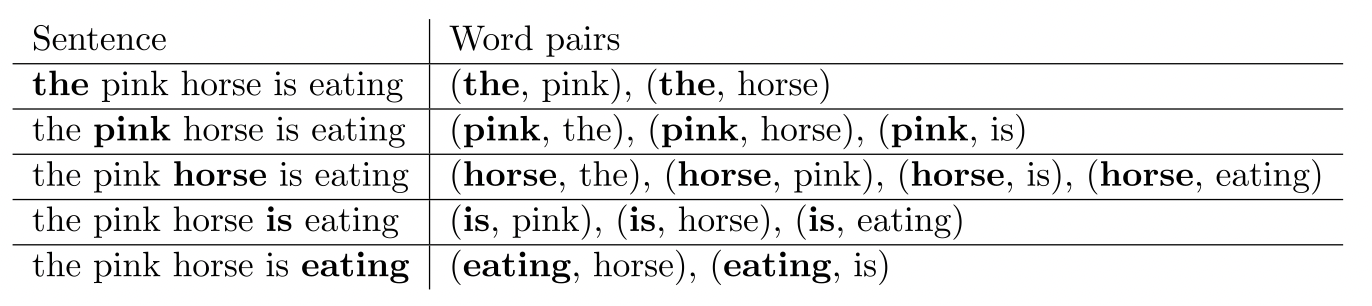With the help of the above table, we can see the word pairs constructed with this method. The highlighted word denotes the word for which we want to find pairs. Here, we don’t care about how much the distance between the words in the window is. As long as words are inside the window, we don’t differentiate between words that are 1 word away or more.

### The General Flow of the Algorithm

• Step-1: Initially, we will assign a vector of random numbers to each word in the corpus.
• Step-2: Then, we will iterate through each word of the document and grab the vectors of the nearest n-words on either side of our target word, and concatenate all these vectors, and then forward propagate these concatenated vectors through a linear layer + softmax function, and try to predict what our target word was.
• Step-3: In this step, we will compute the error between our estimate and the actual target word and then backpropagated the error and then modifies not only the weights of the linear layer but also the vectors or embeddings of our neighbor’s words.
• Step-4: Finally, we will extract the weights from the hidden layer and by using these weights encode the meaning of words in the vocabulary.

Word2Vec model is not a single algorithm but is composed of the following two preprocessing modules or techniques:

• Continuous Bag of Words (CBOW)
• Skip-Gram.

Both of the mentioned models are basically shallow neural networks that map word(s) to the target variable which is also a word(s). These techniques learn the weights that act as word vector representations. Both these techniques can be used to implementing word embedding using word2vec.

Before going further deep dive into the two techniques of Word2Vec, let’s first try to understand the given below question :

### Why Word2Vec technique is created?

As we know that most of the NLP systems treat words as atomic units. In existing systems with the same purpose as that of word2vec, there is a disadvantage that there is no notion of similarity between words. Also, those system works for small, simpler data and outperforms on because of only a few billions of data or less.

So, In order to train the system with a larger dataset with complex models, these techniques use a neural network architecture to train complex data models and outperform huge datasets with billions of words and with vocabulary having millions of words.

It helps to measure the quality of the resulting vector representations and works with similar words that tend to close with words that can have multiple degrees of similarity.

Syntactic Regularities: These regularities refer to grammatical sentence correction.

Semantic Regularities: These regularities refer to the meaning of the vocabulary symbols arranged in that structure.

The proposed technique was found that the similarity of word representations goes beyond syntactic regularities and works surprisingly well for algebraic operations of word vectors.

For Example,

`Vector(“King”) — Vector(“Man”)+Vector(“Woman”) = Word(“Queen”)`

where “Queen” is considered the closest result vector of word representations.

The above new two proposed models i.e, CBOW and Skip-Gram in Word2Vec uses a distributed architecture that tries to minimize the computation complexity.

## Continuous Bag of Words (CBOW)

The aim of the CBOW model is to predict a target word in its neighborhood, using all words. To predict the target word, this model uses the sum of the background vectors. For this, we use the pre-defined window size surrounding the target word to define the neighboring terms that are taken into account.

### Case-1: Single context word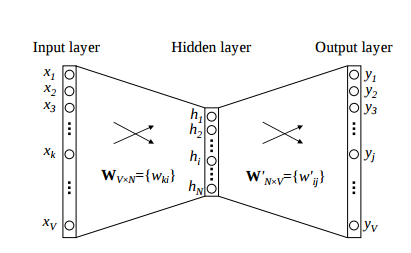We breakdown the way this model works in the following steps:

1. Firstly, the input layer and the target, both are one-hot encoded of size [1 X V].
2. In this model, we have two sets of weights- one is between the input and the hidden layer and the second between the hidden and output layer.
3. Input-Hidden layer matrix size =[V X N], hidden-Output layer matrix size = [N X V]: Where N is an arbitrary size that defines the size of our embedding space or the number of dimensions that we choose to represent our word in. It is a hyper-parameter for a Neural Network. Also, N is the number of neurons present in the hidden layer.
4. There is no activation function present between any of the layers in the model or More specifically, we can refer to this as a linear activation.
5. The input is multiplied by the weights present between the input and hidden layer and it is known as hidden activation. It becomes the corresponding row in the input-hidden matrix copied.
6. The hidden input gets multiplied by weights present between hidden and output layers and output is computed.
7. Then, compute the error between output and target and using that error propagated back to re-adjust the weights upto we achieve the minimum error.
8. So, the weight between the hidden layer and the output layer is taken as the word vector representation of the word.

### Case-2: Multiple context words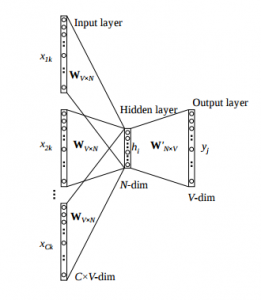Let’s consider the following matrix representation for a specified example: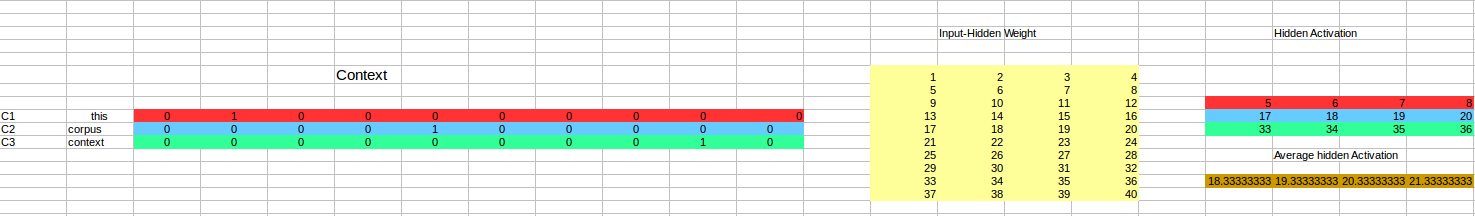As we can observe in the above image, it takes 3 context words and predicts the probability of a target word.

INPUT: The input can be assumed as taking three one-hot encoded vectors in the input layer as shown above in red, blue, and green.

So, the input layer will have 3 [1 X V] Vectors and we have 1 [1 X V] vector in the output layer. The rest of the architecture is the same as for a 1-context CBOW.

The above-mentioned steps remain the same but the only thing that changes is the calculation of hidden activation. Here, instead of just sending the corresponding rows of the input-hidden weight matrix to the hidden layer, an average is taken over all the corresponding rows of the matrix. We can understand this with the above figure. Therefore, the average vector calculated becomes the hidden activation.

So, if for a single target word we have three context words, then we will have three initial hidden activations which we are averaged element-wise to obtain the final activation.

### Objective Function of CBOW Model

The objective function in CBOW is the negative log-likelihood of a word given a set of context i.e -log( p( wo/wi )), where p( wo/wi ) is given as: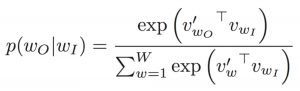where,

wo: output word

wi: context words

1. Generally, it is supposed to perform superior to deterministic methods due to its probabilistic nature.

2. It does not need to have huge RAM requirements. So, it is low on memory.

1. CBOW takes the average of the context of a word. For Example, consider the word apple that can be both a fruit and a company but CBOW takes an average of both the contexts and places it in between a cluster for fruits and companies.

2. If we want to train a CBOW model from scratch, then it can take forever if we not properly optimized it.

### Homework Problem

Do you think that Multi-layer Perceptrons (MLP) is the same as of CBOW model? If not, examine the differences between these two models based on Objective function and Error Gradient.

## Skip-Gram

1. Given a word, the Skip-gram model predicts the context.

2. Skip–gram follows the same topology as CBOW. It just flips CBOW’s architecture on its head. Therefore, the skip-gram model is the exact opposite of the CBOW model.

3. In this case, the target word is given as the input, the hidden layer remains the same, and the output layer of the neural network is replicated multiple times to accommodate the chosen number of context words.

### General Steps involved in the algorithm

1. Let’s the input vector give to a skip-gram is going to be similar to a 1-context CBOW model. Note that the calculations up to hidden layer activations are going to be the same.
2. The difference will be in the target variable. Since we have defined a context window of 1 on both sides of our target word, we will be getting “two” one-hot encoded target variables and “two” corresponding outputs which are represented by the blue section in the below image.
3. Then, we compute the two separate errors with respect to the two target variables, and then we add the two error vectors element-wise to obtain a final error vector which is propagated back to update the weights until our objective is met.
4. Finally, the weights present between the input and the hidden layer are considered as the word vector representation after training. The loss function or the objective is of the same type as the CBOW model.

Now, let’s see the architecture of the skip-gram model: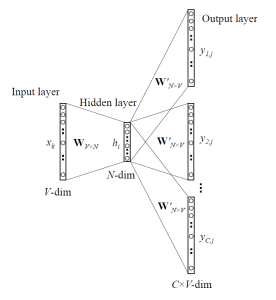For a better understanding, let’s see the matrix-style structure given below: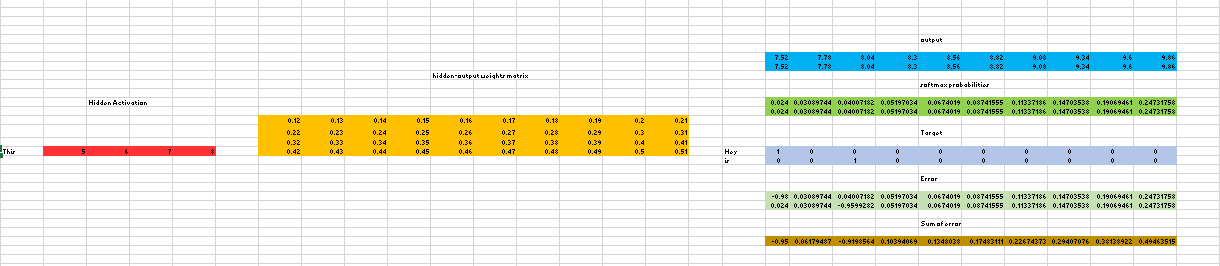We breakdown the way this model works in the following steps:

For the above matrix, the sizes of different layers are as follows:

• Size of Input layer ——— [1 X V],
• Size of Input hidden weight matrix ———– [V X N],
• Number of neurons present in the hidden layer —— N,
• Size of Hidden-Output weight matrix ——- [N X V],
• Size of Output layer —- C [1 X V]

In the above example, C is the number of context words=2, and V= 10, N=4.

1. The red row represents the hidden activation corresponding to the input one-hot encoded vector. It basically represents the corresponding row of the input-hidden matrix.
2. The yellow matrix is the weights present between the hidden layer and the output layer.
3. To obtain the blue matrix, we do the matrix multiplication of hidden activation and the hidden output weights, and there will be two rows calculated for two targets (context) words.
4. Then, we convert each row of the blue matrix into its softmax probabilities individually which is shown in the green box.
5. Here, the grey matrix describes the one-hot encoded vectors of the two context words i.e, target.
6. Error is calculated by subtracting the first row of the grey matrix(target) from the first row of the green matrix(output) element-wise. This is repeated for the next row. Therefore, if we have n target context words, then we will have n error vectors.
7. The element-wise sum is taken over all the error vectors to obtain a final error vector.
8. Finally, the calculated error vector is backpropagated to adjust the weights.

1. The Skip-gram model can capture two semantics for a single word. i.e two vector representations for the word Apple. One for the company and the other for the fruit.

2. Generally, Skip-gram with negative sub-sampling performs well then every other method.

## Tool for Visualize CBOW and Skip-Gram

To visualize CBOW and skip-gram in action, the given below is a very excellent interactive tool. I would suggest you really go through this link for a better understanding.

Visualize CBOW and Skip-Gram

## When to use which- CBOW or Skip-Gram?

Now that we have a broad idea of both the models involved in the Word2Vec Technique, which one is better? Of course, which model we choose from the above two largely depends on the problem statement we’re trying to solve.According to the original paper, Mikolov et al., it is observed that the Skip-Gram model works well with a small amount of the training datasets, and can better represent rare words or phrases.

However, the CBOW model is observed to train faster than Skip-Gram, and can better represent more frequent words which mean gives slightly better accuracy for the frequent words.

### Conclusion

1. Is it important for our model to represent rare words? If so, we should choose Skip-Gram since when their vectors are not averaged with the other background terms in the process of making the predictions, the model will learn better representations for the rare words and it is better to use the Skip-gram model in that case.

2. We don’t have much time to train and rare words are not that important for our solution? Then we should choose CBOW.

In the end, since different applications have distinct criteria, the best practice is to try a few tests to see what works best for you.

## Project Idea to use Word Embeddings for Text Classification

The objective of the given problem statement is to detect hate speech in tweets. In simple words, we can say a tweet contains hate speech if it has a racist or sexist sentiment associated with it. So, now our task is to identify the racist or sexist tweets and classify also from other tweets.

We have given a training sample containing tweets and labels, where

• label ‘1’ denotes the tweet is racist/sexist
• label ‘0’ denotes the tweet is not racist/sexist,

Your objective is to predict the labels on the unseen or test dataset.

To practice the above problem statement, open the given link and start now.

## Other Blog Posts by Me

You can also check my previous blog posts.

Previous Data Science Blog posts.

Here is my Linkedin profile in case you want to connect with me. I’ll be happy to be connected with you.

## Email

For any queries, you can mail me on .

## End Notes